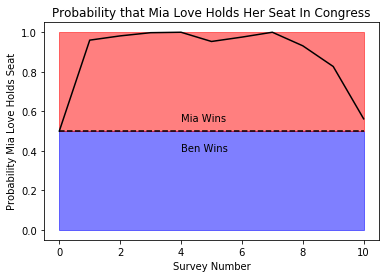### Bayes’ Rule and Combining Data From Different PollsIn my last blog post, I asked the question, “Can Mia Love hold her congressional seat?” And based off of a single poll, the answer was grim for Mia Love, there is only a one in five shot that she’ll be able to pull off the win. Now, I don’t want to be a naysayer for Mia Love. So I want to give her the benefit of the doubt. In this post, I combine a few more polls. And since it is election day, I thought, let’s see what we can do.

That’s why in this post, I want to look at more than just the most recent poll. I want to look at how she has been doing overall. I think that is very important as well. So if there were only some way that we could combine evidence from different polls to get at our answer. Oh wait, there is. It’s called Bayes Rule/Theorem. I’ve talked about it before. Anyway, what we’re going to do in this post is basically take the last 10 polls from different organizations and combine them into a single number.

So I just grabbed the results of those polls and their margin of errors (it turns out they all used 5 point margin of errors which made the coding to follow slightly easier). At any rate, what we want to know, is where do we think Mia Love’s probability of holding her seat sits, given the information in the polls. So we start by assuming that before we have any polling data, the probability is 50/50. I know that isn’t necessarily true, but it is the most fair way to split that probability initially, and it sort of neglects any incumbency effect, giving Ben McAdams a fair shake with our algorithm.

Anyway, we calculate the probability that Mia Love will win the election in each poll and then naively apply Bayes theorem to our prior. We then use the updated probability as our new prior, and apply Bayes theorem again using the next poll, and so on until we run out of polls. When you do that you get a sequence of probabilities, that you could plot over time. So that’s what I did. This is what that produces.

It looks like despite the recent dip, Mia is more or less guaranteed the win. Sorry Ben, looks like this isn’t going to be your year. What’s that, I’m ignoring time? Okay, well, let’s mess around with it a little bit more.

Let’s add a fudge factor to the model. In the code, I call it magic because when you add in the fudge factor, you can basically make the model say whatever you want, within a certain limit. So how does my fudge factor work? It takes a page out of economics and discounts older polls more heavily than newer polls. Essentially, we’re telling Bayes theorem to pay more attention to recent polls, and kind of ignore the older polls. Now how strongly you ignore the older polls is the bit of magic. You can set that really high. In which case you only count the most recent poll, or really low, which is basically what you get from a naive approach. I settled in on a value of 0.07 for my magic number, mostly by playing with the magic number and figuring out what would give me a value that reflects the kind of uncertainty that this number introduces by varying it to crazy extreme values. I also like that number because it jives with what I’ve used in economics. It is about as myopic as humans tend to be, so I am pleased that number popped out.

Here is the code that I used:

# -*- coding: utf-8 -*-
"""
Created on Fri Oct 26 10:24:35 2018

@author: rbarnes
"""

import matplotlib.pyplot as plt
import scipy.stats as s
import numpy as np
result = 0.5
probs = [0.5]
polls = [0.03,0.04,0.06,0.09,0.02,0.03,0.09,0.00,-0.01,-0.02]
magics = [0.0,0.07373]
for magic in magics:
probs=[0.5]
i=0
for poll in polls:
i+=1
#factor = result
factor = (1-np.exp(-magic*(len(polls)-i)))*0.5+np.exp(-magic*(len(polls)-i))*result
probs.append((1-s.norm(loc=poll, scale=0.025).cdf(0))*factor/((1-s.norm(loc=poll, scale=0.025).cdf(0))*factor+(s.norm(loc=poll, scale=0.025).cdf(0))*(1-factor)))
print(result)
result = (1-s.norm(loc=poll, scale=0.025).cdf(0))*factor/((1-s.norm(loc=poll, scale=0.025).cdf(0))*factor+(s.norm(loc=poll, scale=0.025).cdf(0))*(1-factor))

plt.plot(range(len(probs)),probs,'k')
plt.fill_between(range(len(probs)),0.5,color='b',alpha=0.5)
plt.fill_between(range(len(probs)),y1=1.0,y2=0.5,color='r',alpha=0.5)
plt.hlines(0.5,0,10,linestyle='--',colors='k')
plt.text(4,0.4,'Ben Wins')
plt.text(4,0.55,'Mia Wins')
plt.ylabel('Probability Mia Love Holds Seat')
plt.xlabel('Survey Number')
plt.title('Probability that Mia Love Holds Her Seat In Congress')
plt.show()
print(result)

That resulted in this image,  which suggests that the race really is a toss up at the moment. Mia Love has a slight edge at holding her seat at about 56% probability. But notice how her standing has slid heavily in the last few weeks. Four polls ago, it was pretty much a lock for Mia Love, but now she needs to get out there and fight, because there is a solid chance Ben McAdams will take her down. Plus the trend doesn’t look good. Notice the steep decline in probability of her retaining her seat, will that trend reverse? I mean it is one thing to see a gradual decline, but this was a fall off of a cliff, her numbers look terrible. I suspect that she need to turn this around quickly, or she won’t be going back to DC next year.

All of the code is also available on my github as well. If you noticed the timestamp on this is current as of October 26th. Newer polls haven’t been taken into account. Mia has slipped a bit more, but held at around a 43% probability of keeping her seat. This one should be a nail biter for sure.

Anyway, get out and vote! And good luck to all of our candidates.